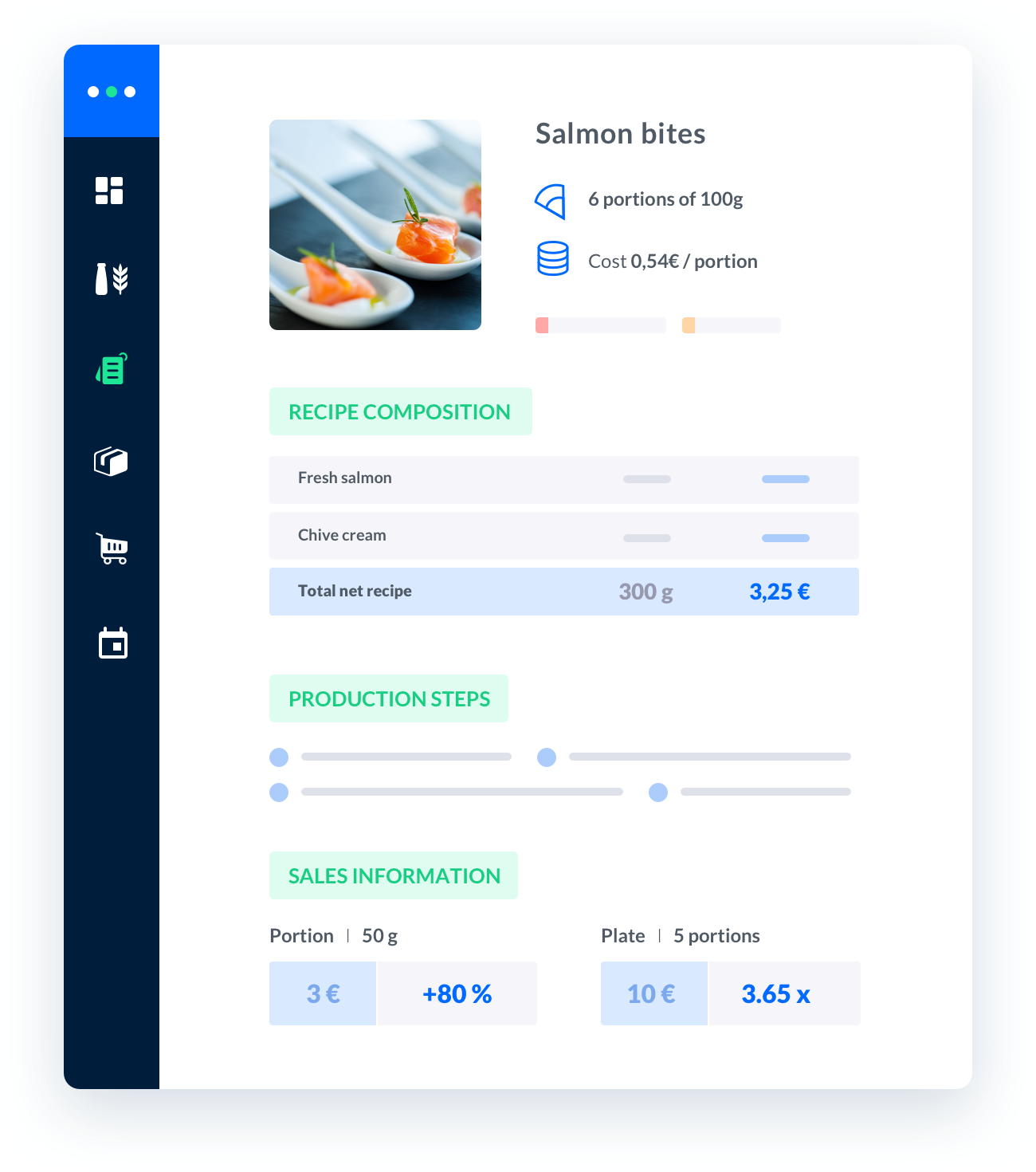"When it comes to running a successful bakery or catering business, it is crucial to have a clear understanding of your expenses and profit margins.One of the most effective tools to help you calculate your baking costs is a spreadsheet.

Published on 01/01/2023Sébastien Vassaux##### Melba: the food cost app to optimize the profitability of your restaurant

Discover how to optimize the profitability of your restaurant with melba"

## The Significance of a Baking Cost Calculator Spreadsheet

A baking cost calculator spreadsheet serves as a valuable resource for any catering professional. It enables you to track and analyze your expenses, ensuring that you are aware of the true cost of producing each baked item. Here are some key reasons why a baking cost calculator spreadsheet is essential:

• Accurate Pricing: By calculating the precise cost of ingredients, packaging, and other expenses, you can set appropriate prices for your baked goods. This ensures that you are not undercharging or overcharging customers, maximizing your profitability.
• Cost Control: With a baking cost calculator spreadsheet, you can identify areas where you can reduce costs without compromising the quality of your products. This helps you maintain a healthy profit margin and optimize your business operations.
• Decision Making: Knowing the exact cost of each item allows you to make informed decisions regarding menu changes, ingredient substitutions, and special promotions. This helps you evaluate the financial impact of such decisions before implementing them.

### Step 1: Set Up the Spreadsheet

Start by opening a blank spreadsheet in your preferred software, such as Microsoft Excel or Google Sheets. Name the spreadsheet and create columns for the following categories:

• Item: List the name or description of each baked item.
• Quantity: Enter the quantity of each ingredient required for the recipe.
• Unit Cost: Specify the cost of each ingredient per unit (e.g., per pound, per ounce).
• Total Cost: This column will automatically calculate the total cost of each ingredient by multiplying the quantity with the unit cost.
• Other Expenses: Include any additional expenses related to packaging, labor, or overhead costs.
• Total Cost per Item: This column will sum up the total ingredient cost and other expenses, giving you the overall cost of producing each item.

### Step 2: Input Ingredient Costs

Begin entering the ingredients required for each baked item in the ""Item"" column. Then, input the quantity and unit cost for each ingredient in their respective columns. The spreadsheet will automatically calculate the total cost for each ingredient and display it in the ""Total Cost"" column.

### Step 3: Add Other Expenses

Consider any additional expenses associated with producing each baked item. This may include costs for packaging materials, labor, utilities, or rent. Enter these expenses in the ""Other Expenses"" column.

### Step 4: Calculate Total Cost per Item

In this step, the spreadsheet will calculate the total cost per item by summing up the total ingredient cost and other expenses. Use a formula (e.g., ""=SUM(Total Cost + Other Expenses)"") to automate this calculation for each item.

## Best Practices for Using a Baking Cost Calculator Spreadsheet

To ensure the effectiveness of your baking cost calculator spreadsheet, consider the following best practices: